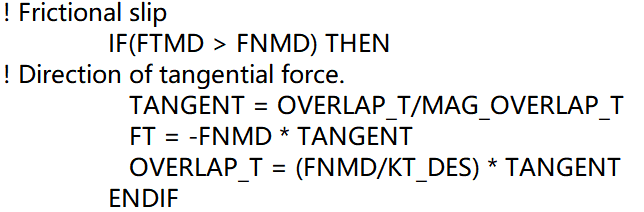Questions on tangential overlap calculation in DEM

Hi, every one. I want to implement Burgers model in DEM. The illustration of the model shows as follows. It is the combination of Maxwell and Kelvin model.

I want to know how to calculate the tangential overlap of the dash pot in the middle when two particles are sliding. All model parameters, like, K, C and friction coefficient, are known.

By the way, the original tangential overlap of two sliding particles is calculated as follows. I wonder why the viscous force is not subtracted from the FT and then divided by the spring elastic coefficient.